# Interpret the key results for Poisson Capability Analysis

Complete the following steps to interpret Poisson Capability Analysis. Key output includes the control chart, the Poisson plot, and the mean DPU.

## Step 1: Determine whether your process is stable

Your process should be stable to obtain reliable estimates of process capability.

Red points indicate subgroups that fail at least one of the tests for special causes and are not in control. Out-of-control points indicate that the process may not be stable and that the results of a capability analysis may not be reliable. You should identify the cause of out-of-control points and eliminate special-cause variation before you analyze process capability.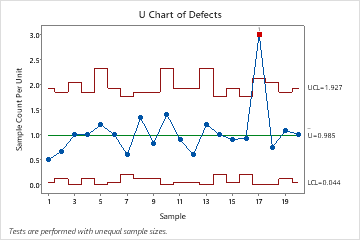In this U chart, most of the points vary randomly and are within the control limits. No trends or patterns are present. However, the rate of defects per unit for day 17 is out of control. Before you evaluate the capability of the process, investigate and eliminate any special causes that may have contributed to the unusually high DPU on that day.

## Step 2: Determine whether the data follow a Poisson distribution

Before you evaluate the capability of your process, determine whether it follows a Poisson distribution. If your data do not follow a Poisson distribution, the estimates of process capability may not be reliable. The graph that Minitab displays to evaluate the distribution of the data depends on whether your subgroup sizes are equal or unequal.

### Subgroup sizes are equal

If your subgroup sizes are all the same, Minitab displays a Poisson plot.

Examine the plot to determine whether the plotted points approximately follow a straight line. If not, then the assumption that the data were sampled from a Poisson distribution may be false.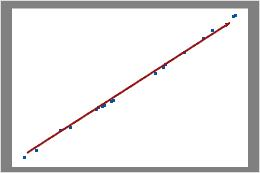PoissonIn this plot, the data points fall closely along the line. You can assume that the data follow a Poisson distribution.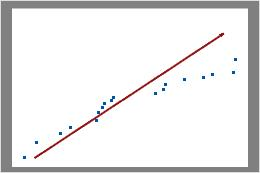Not PoissonIn this plot, the data points do not fall along the line at the top right of the plot. These data do not follow a Poisson distribution and cannot be reliably evaluated using Poisson capability analysis.

### Subgroup sizes are not equal

If the subgroup sizes vary, Minitab displays a defect rate plot.

Examine the plot to assess whether the defects per unit (DPU) are randomly distributed across sample sizes or whether a pattern is present. If your data fall randomly about the center line, you conclude that the data follow a Poisson distribution.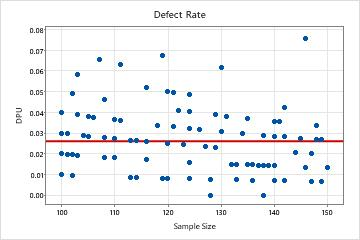PoissonIn this plot, the points are scattered randomly around the center line. You can assume that the data follow a Poisson distribution. Therefore, the data can be evaluated using Poisson capability analysis.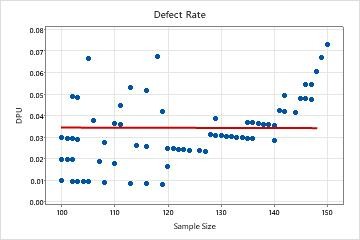Not PoissonIn this plot, the pattern is not random. For sample sizes greater than 120, DPU increases as the sample size increases. This result suggests a possible correlation between sample size and the rate of defects. Therefore, the data do not follow a Poisson distribution and cannot be reliably evaluated using Poisson capability analysis.

## Step 3: Evaluate the mean defects per unit (DPU)

### Examine the Mean DPU estimate and CI

Use the mean DPU of the sample data to estimate the mean DPU of the process. Use the confidence interval as a margin of error for the estimate.

The confidence interval provides a range of likely values for the actual value of the mean DPU in your process (if you could collect and analyze all of the items it produces). At a 95% confidence level, you can be 95% confident that the actual mean DPU of the process is contained within the confidence interval. That is, if you collect 100 random samples from your process, you can expect approximately 95 of the samples to produce intervals that contain the actual value of mean DPU.

The confidence interval helps you to assess the practical significance of your sample estimate. If you have a maximum allowable mean DPU value that is based on process knowledge or industry standards, compare the upper confidence bound to this value. If the upper confidence bound is less than the maximum allowable mean DPU value, then you can be confident that your process meets specifications, even when taking into account variability from random sampling that affects the estimate.

Summary Stats
(95.0% confidence)
Mean DPU: 0.0225
Lower CI: 0.0190
Upper CI: 0.0265
Min DPU: 0.0000
Max DPU: 0.0661
Targ DPU: 0.0250

### Determine whether you have enough data for a reliable estimate

Use the Cumulative DPU plot to help you determine whether you have collected enough samples to have a stable estimate of the DPU.

Examine the defects per unit for the time-ordered samples to see how the estimate changes as you collect more samples. Ideally, the DPU should stabilize after several samples, as shown by a flattening of the plotted points along the mean DPU line.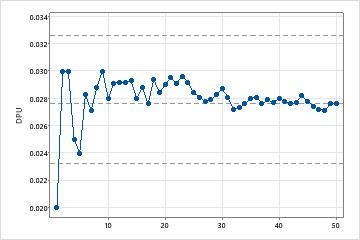Enough samplesIn this plot, the DPU stabilizes along the mean DPU line. Therefore, the capability study includes enough samples to produce a stable, reliable estimate of the mean DPU.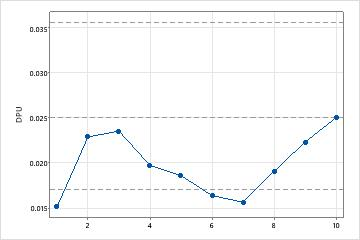Not enough samplesIn this plot, the DPU does not stabilize. Therefore, the capability study does not include enough samples to reliably estimate the mean DPU.